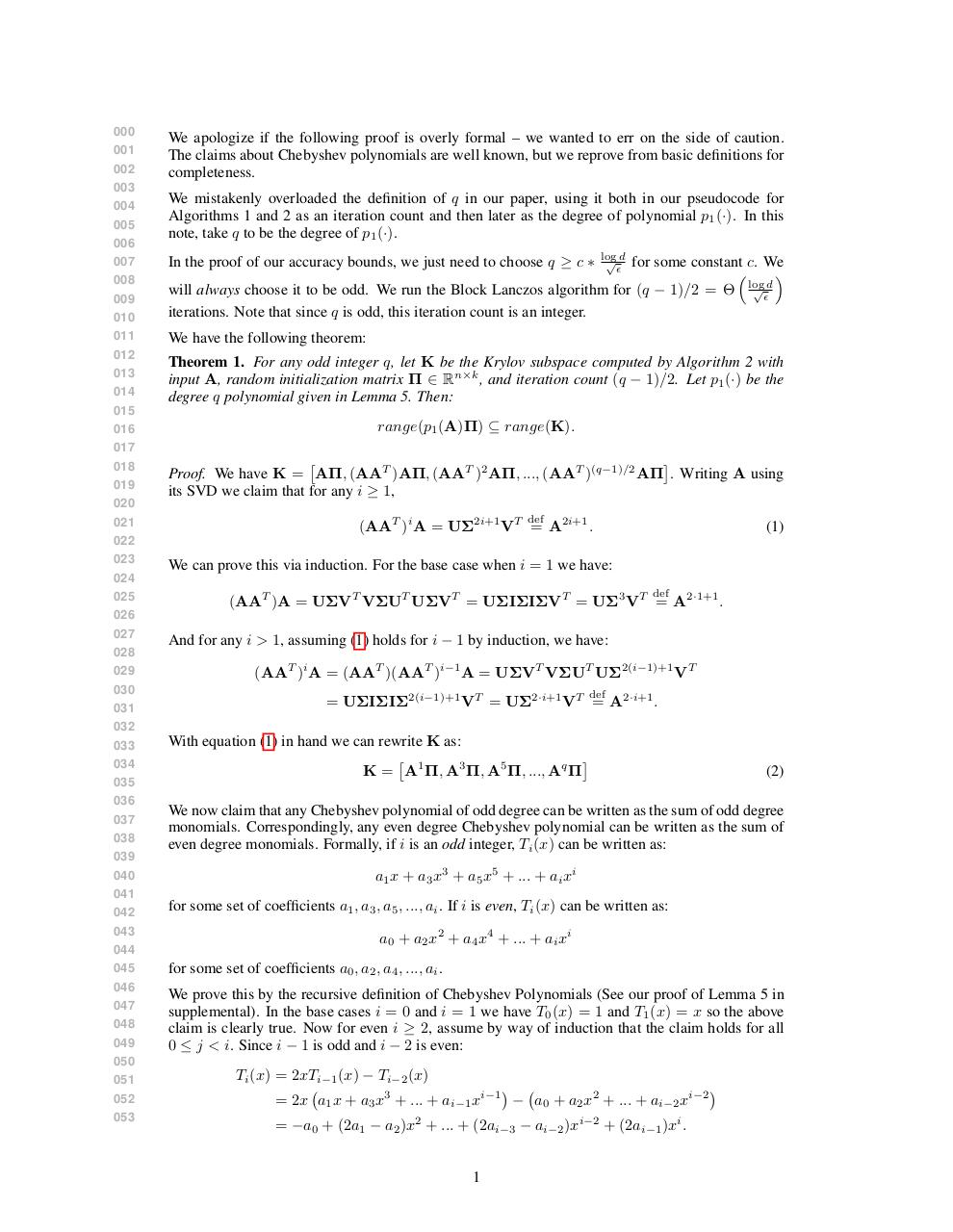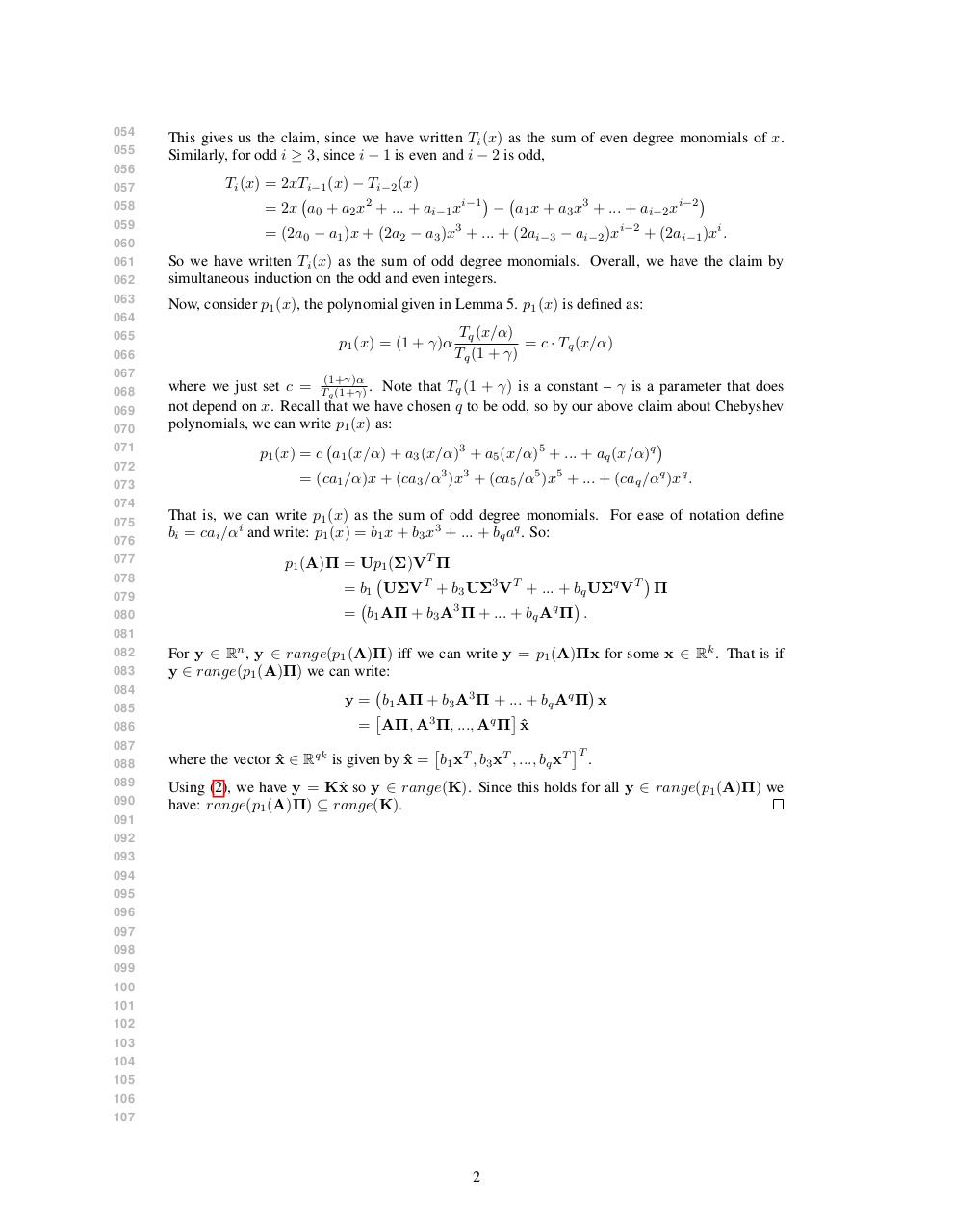# polyProof .pdf

### File information

Original filename: polyProof.pdf

This PDF 1.5 document has been generated by LaTeX with hyperref package / pdfTeX-1.40.15, and has been sent on pdf-archive.com on 07/08/2015 at 14:12, from IP address 209.131.x.x. The current document download page has been viewed 507 times.
File size: 130 KB (2 pages).
Privacy: public file

## Download original PDF file

polyProof.pdf (PDF, 130 KB)

### Document preview

000
001
002
003
004
005
006
007
008
009
010
011
012
013
014
015
016
017
018
019
020
021
022
023
024

We apologize if the following proof is overly formal – we wanted to err on the side of caution.
The claims about Chebyshev polynomials are well known, but we reprove from basic definitions for
completeness.
We mistakenly overloaded the definition of q in our paper, using it both in our pseudocode for
Algorithms 1 and 2 as an iteration count and then later as the degree of polynomial p1 (·). In this
note, take q to be the degree of p1 (·).
In the proof of our accuracy bounds, we just need to choose q ≥ c ∗

log
√d

for some constant c. We

√d
will always choose it to be odd. We run the Block Lanczos algorithm for (q − 1)/2 = Θ log

iterations. Note that since q is odd, this iteration count is an integer.
We have the following theorem:

Theorem 1. For any odd integer q, let K be the Krylov subspace computed by Algorithm 2 with
input A, random initialization matrix Π ∈ Rn×k , and iteration count (q − 1)/2. Let p1 (·) be the
degree q polynomial given in Lemma 5. Then:
range(p1 (A)Π) ⊆ range(K).

Proof. We have K = AΠ, (AAT )AΠ, (AAT )2 AΠ, ..., (AAT )(q−1)/2 AΠ . Writing A using
its SVD we claim that for any i ≥ 1,
def

(AAT )i A = UΣ2i+1 VT = A2i+1 .
We can prove this via induction. For the base case when i = 1 we have:
def

025
026
027
028
029
030

And for any i &gt; 1, assuming (1) holds for i − 1 by induction, we have:

031
032
033
034
035
036

With equation (1) in hand we can rewrite K as:

K = A1 Π, A3 Π, A5 Π, ..., Aq Π

037
038
039
040
041
042
043
044
045
046
047
048
049
050
051
052
053

(1)

(AAT )A = UΣVT VΣUT UΣVT = UΣIΣIΣVT = UΣ3 VT = A2·1+1 .

(AAT )i A = (AAT )(AAT )i−1 A = UΣVT VΣUT UΣ2(i−1)+1 VT
def

= UΣIΣIΣ2(i−1)+1 VT = UΣ2·i+1 VT = A2·i+1 .

(2)

We now claim that any Chebyshev polynomial of odd degree can be written as the sum of odd degree
monomials. Correspondingly, any even degree Chebyshev polynomial can be written as the sum of
even degree monomials. Formally, if i is an odd integer, Ti (x) can be written as:
a1 x + a3 x3 + a5 x5 + ... + ai xi
for some set of coefficients a1 , a3 , a5 , ..., ai . If i is even, Ti (x) can be written as:
a0 + a2 x2 + a4 x4 + ... + ai xi
for some set of coefficients a0 , a2 , a4 , ..., ai .
We prove this by the recursive definition of Chebyshev Polynomials (See our proof of Lemma 5 in
supplemental). In the base cases i = 0 and i = 1 we have T0 (x) = 1 and T1 (x) = x so the above
claim is clearly true. Now for even i ≥ 2, assume by way of induction that the claim holds for all
0 ≤ j &lt; i. Since i − 1 is odd and i − 2 is even:
Ti (x) = 2xTi−1 (x) − Ti−2 (x)

= 2x a1 x + a3 x3 + ... + ai−1 xi−1 − a0 + a2 x2 + ... + ai−2 xi−2
= −a0 + (2a1 − a2 )x2 + ... + (2ai−3 − ai−2 )xi−2 + (2ai−1 )xi .
1

054
055
056
057
058
059
060
061
062
063
064
065
066
067
068
069
070
071
072
073
074
075
076
077
078
079
080
081
082
083
084
085
086
087
088
089
090

This gives us the claim, since we have written Ti (x) as the sum of even degree monomials of x.
Similarly, for odd i ≥ 3, since i − 1 is even and i − 2 is odd,
Ti (x) = 2xTi−1 (x) − Ti−2 (x)

= 2x a0 + a2 x2 + ... + ai−1 xi−1 − a1 x + a3 x3 + ... + ai−2 xi−2
= (2a0 − a1 )x + (2a2 − a3 )x3 + ... + (2ai−3 − ai−2 )xi−2 + (2ai−1 )xi .
So we have written Ti (x) as the sum of odd degree monomials. Overall, we have the claim by
simultaneous induction on the odd and even integers.
Now, consider p1 (x), the polynomial given in Lemma 5. p1 (x) is defined as:
p1 (x) = (1 + γ)α

Tq (x/α)
= c · Tq (x/α)
Tq (1 + γ)

where we just set c = T(1+γ)α
. Note that Tq (1 + γ) is a constant – γ is a parameter that does
q (1+γ)
not depend on x. Recall that we have chosen q to be odd, so by our above claim about Chebyshev
polynomials, we can write p1 (x) as:

p1 (x) = c a1 (x/α) + a3 (x/α)3 + a5 (x/α)5 + ... + aq (x/α)q
= (ca1 /α)x + (ca3 /α3 )x3 + (ca5 /α5 )x5 + ... + (caq /αq )xq .
That is, we can write p1 (x) as the sum of odd degree monomials. For ease of notation define
bi = cai /αi and write: p1 (x) = b1 x + b3 x3 + ... + bq aq . So:
p1 (A)Π = Up1 (Σ)VT Π

= b1 UΣVT + b3 UΣ3 VT + ... + bq UΣq VT Π

= b1 AΠ + b3 A3 Π + ... + bq Aq Π .
For y ∈ Rn , y ∈ range(p1 (A)Π) iff we can write y = p1 (A)Πx for some x ∈ Rk . That is if
y ∈ range(p1 (A)Π) we can write:

y = b1 AΠ + b3 A3 Π + ... + bq Aq Π x

= AΠ, A3 Π, ..., Aq Π x
ˆ

T
where the vector x
ˆ ∈ Rqk is given by x
ˆ = b1 xT , b3 xT , ..., bq xT .
Using (2), we have y = Kˆ
x so y ∈ range(K). Since this holds for all y ∈ range(p1 (A)Π) we
have: range(p1 (A)Π) ⊆ range(K).

091
092
093
094
095
096
097
098
099
100
101
102
103
104
105
106
107

2### Link to this page

#### Permanent link

Use the permanent link to the download page to share your document on Facebook, Twitter, LinkedIn, or directly with a contact by e-Mail, Messenger, Whatsapp, Line..

#### Short link

Use the short link to share your document on Twitter or by text message (SMS)

#### HTML Code

Copy the following HTML code to share your document on a Website or Blog

#### QR Code### Related keywords